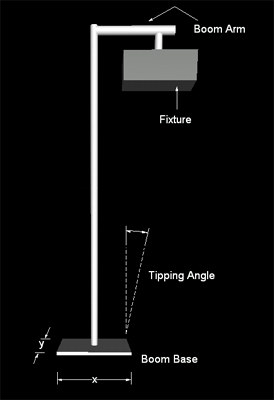# Robin Barton

### General

Beaufort Wind Scale
Convert Units
IP Rating

### Electronics

Basic Calculations
Resistor Calculator
Resistor Circuits
Resistor Colour Code

### Mechanical

Centre of Gravity
Centre of Mass

# Centre of Mass

The centre of mass of an object is the point at which the mass of that object is effective. For a regular shaped piece of solid uniform material, that point is in the geometric centre, for example:

• On a sheet of paper draw a line from each corner to the diagonally opposite corner so that you end up with a cross. The centre of mass is where the lines cross and halfway through the thickness of the paper.
• The centre of mass of a 2m x 2m x 2m cube is at 1m,1m,1m.
• The centre of mass of a sphere is in the centre.

## Determining the Centre of Mass

Most objects are not regular shaped or uniform material. This leave us with two options: calculation or practical determination.

### Practical Determination

A very simple method is to hang the object in question from a single point. Attach a plumb line to the hanging point and draw a line on the object along the plumb line. Hang the item from a different point, attach the plumb line to that point and draw another line. The centre of mass is at the point that the two lines intersect. If you need the centre of mass in three dimensions, turn the object 90° and repeat the steps above.

### Calculation the Centre of Mass

The easiest way to calculate the centre of mass of a complex object is to split it into component parts of regular shapes. The properties of each shape are then combined summing the products of mass and centre of mass co-ordinates of each section and dividing the total by the total

#### Example 1

Diagram of 'L' shape 10m tall 8m wide and 3m thick split into 10 x 3 and 5 x 3 and 1m deep.

The shaped above is made of a uniform material with a mass of 1kgm-3

• Split the object into regular section:
A = 3m x 10m x 1m, B = 5m x 3m x 1m.
• Determine the position of the centre of mass of each section:
CoMA = (1.5m,5m,0.5m)
CoMB = (5.5m,1.5m,0.5m)
• Caclulate the mass of each section:
MA = 3 x 10 x 1 * 1 = 30kg
MB = 5 x 3 x 1 = 15kg
• Caclulate the overall centre of Mass: CoMx = (CoMAx x MA + CoMBx x MB) / (MA + MB)
CoMx = (1.5 x 30 + 5.5 x 15) / (30 + 15) = 127.5 / 45 = 2.83
CoMy = (5 x 30 + 1.5 x 15) / (30 + 15) = 172.5 / 45 = 3.83
CoMz = (0.5 x 30 + 0.5 x 15) / (30 + 15) = 22.5 / 45 = 0.5
Thus CoM = (2.83,3.83,0.5)

#### Example 2

A 0.5m boom arm is rigged at 2.5m high with a 10kg lighting fixture rigged at 0.4m from the centre of the upright pole. In this example, we will use the centre of the bottom of the boom base as our origin.

Adujst the values below to recalculate the Centre of Mass

 Upright Scaffold TubeBoom Arm Material AlloySteel Material AlloySteel Height Length Mass kg Mass kg Centre of Mass Height m Boom base Centre of Mass Width (x) m Fixture Depth (y) m Hanging Position m Thickness (z) m Mass kg Mass kg Centre of Mass Centre of Mass This is assumed to be 0.2mdirectly under the hanging point. Overall System Mass 20.295 kg Overall System Centre of Mass 0.15, 0.00, 1.64 Tipping Angle (x) 12° (y) 17°